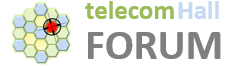# How is CFi related to Code Rate and link Quality of the LTE network?

Hi guys, Im trying to understand the relation between CFi and Code Rate and Quality of the network?

like if I have bad quality then we need to decrease CFi? what about Code Rate we need to increase?

About highy quality , if we have high quality then we need to increase CFi? what about Code Rate we also need to increase?

I need to understand the implicitly logic between CFi , Code Rate , Quality.

CFI is control format indicator which provides the number of symbols used for PDCCH out of 14 symbols. General values for CFI are 1,2 and 3. With CFI we can calculate the total PDCCH CCE. If our quality is bad means we have to increase the PDCCH CCE aggregation level and we need more PDCCH symbols to accommodate users in one TTI. So we have to increase the CFI in poor radio quality.

2 Likes

What do you mean by CCE aggregation level and CCE? … did the aggregation level means modulation scheme and coding scheme -implicitly what’s found on phy layer-?
is CCE resource element of the greed?

Moreover, if I understand you well … we need to decrease CFI in order to give more PDCCH symbols to the users no? because if CFi=3 and we decrease it to 1 then in lte the total symbols we have is 14 - 1 = 13 and we were having before decreasing the CFi 14-3=11 so at the end we have 13 ofdm symbols which it’s good for our case because we have bad quality . does what I understand correct? because we have bad quality then we must concern to give the user more OFDM symbols for the uE as it’s having bad quality , the maximum OFDM symbols in lte is 14 symbols so we need to maximize the OFDM symbols for the users when it has bad quality and we do that be decrease CFi in order to have maximum OFDM symbols .

Am I right?

thanks alot

What I’m trying to understand is what’s the relation between increasing/decreasing cfi to code rate.
If I have bad quality then we need to increase cfi …what about code rate …should we increase or decrease it because I have bad quality?

Appreciate for explanation the relation between increasing/decreasing cfi and how it affects code rate and what should we do in order to keep code rate compatiable to the new value of cfi that we changed it because bad/good quality.

The question is not clear whether you mean code rate for PDCCH or PDSCH. I will assume you are talking about PDSCH

CFI defines how the 14 symbols are divided in the subframe between PDCCH and PDSCH. Assume 1 resource block (12 subcarriers and 14 symbols), it has 12×14 = 168 resource elements (REs)

If CFI = 1, the RB of PDSCH has 12 × (14-1) = 156 REs. Assume 2x2 MIMO, 12 of them are used vy CRS. Assume 64 QAM, each RE carriers 6 bits so the RB carries (156-12) x 6 = 864bits.
Assume the transport Block Size (TBS) = 616 bits and CRC = 24 bits, the code rate = (616 + 24) / 864 = 0.74

If CFI is increased to 2, the code rate = (616 + 24) / ( ((12 x 12 ) -12)x 6 ) = 0.8

If CFI is increased to 3, the code rate = (616 + 24) / ( ((12 x 11) -12)x 6 ) = 0.89

In case of bad quality, you need lower code rate which is typically done by increasing the resource blocks or reducing modulation. CFI is not typically reduced to improve code rate because smaller CFI means less UEs can be scheduled

1 Like

This is not correct.
There are 2 things. if UE is is very bad quality, it cannot decode the DL control/Data signal with higher code rate.
To download the PDSCH, UE forst need to decode PDCCH, which is done by reading CCEs in PDCCH. If UE is in bad quality, PDCCH should be sent through more CCEs to keep reduced code rate of PDCCH. Hence if there are many UEs with band quality, PDCCH symbols will increase because required CCEs will increase.
Now for PDSCH, it also required to send through lower cade rate. In this case , eMB will either reduce MCS or increase the scheduled RBs or both. Ideally eNB scheduler assign best MCS as per UEs DL quality, then adjust the schedueld RBs.
So when there are many poor RF UEs in network, PDCCH symbol increases, and RB utilization also increased.

Code rate is simply information bits divided by total bits.
Total bits includes information bits, CRC bits and error correction bits.
If the RF condition is bad, we required more CRC, error correction bits in order to decode thansport block.
This reduced number of information bits transmitted. So Code rate also reduced.

1 Like

Well explained👍

RE is the basic resource element in frequency/time domain.
REs are grouped into REG and REGs are grouped into CCEs.
If the radio quality is poor then CCE consumption will be more to provide more robustness for control information.